You are now at: / Support / Glossary /

## Garp3 Icons

If an icon is greyed out, it means the button is inactive in this context.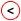Conditional smaller than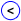Consequence smaller than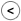Imported smaller than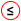Conditional smaller or equal than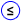Consequence smaller or equal than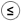Imported smaller or equal than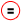Conditional equal to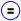Consequence equal to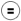Imported equal to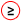Conditional larger or equal to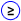Consequence larger or equal to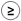Imported larger or equal to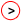Conditional larger than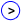Consequence larger than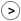Imported larger than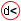Conditional derivative smaller than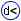Consequence derivative smaller than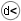Imported derivative smaller than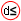Conditional derivative smaller or equal than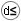Imported derivative smaller or equal than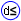Consequence derivative smaller or equal than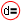Conditional derivative equal to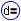Consequence derivative equal toConditional derivative larger or equal to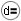Imported derivative equal to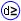Consequence derivative larger or equal to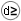Imported derivative larger or equal toConditional derivative larger thanConsequence derivative larger thanImported derivative larger than